# xtlang types

It’s not really possible to explain Extempore’s types without a detour into some memory stuff, so we’ll cover some of that as well. For more info on how xtlang fits into the big picture of Extempore, see philosophy.

xtlang code is statically typed—the types are determined at compile time, and the compiler checks that the types of the arguments to a function match the type signature of that closure. If they don’t match, you get a compile error, and a (hopefully) helpful message about where things are going wrong and what needs to be fixed.

The base types will be familiar to those who are used to working with C, although there are a couple of slight differences. Just like C, the types are all low level in the sense that they represent a particular bit pattern in memory—there’s no boxing and unboxing going on.

In xtlang, type annotations (a type definition following a colon in the source code) are used to tell the compiler about the types of variables. The compiler will infer incomplete type information (such as the size of int and float literals), but will never silently coerce the types. If there’s a problem, the compiler will complain and you have to fix it, either with an explicit coercion or by changing the structure of the code.

The examples here use a lot of these type annotations. This is for clarity—some of these aren’t strictly necessary (as I’ll show later) because the type inferencing compiler will figure out the types of variables in many cases.

## Primitive types

### Integers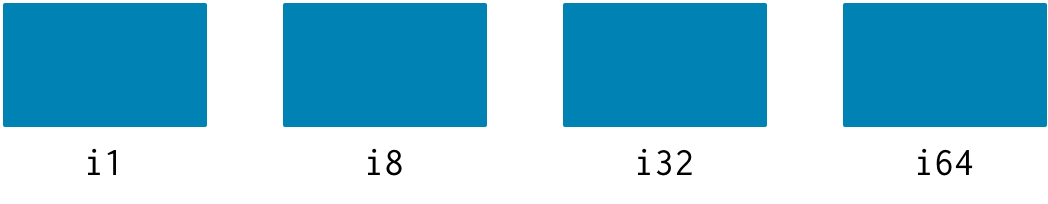Extempore supports signed integers of various different sizes:

• `i1` (boolean)
• `i8` (char)
• `i32`
• `i64` (default)

A couple of gotchas:

• the default integer size is `i64`, so int literals in the code will be interpreted as `i64`, unless the function signature suggests otherwise e.g. if the signature is `[double,i32]*`, then a (single) int literal argument will be read as an `i32`.
• there is no `char` type, only `i8`. In xtlang, strings are null-terminated char arrays/pointers with the signature `i8*`.

### Floats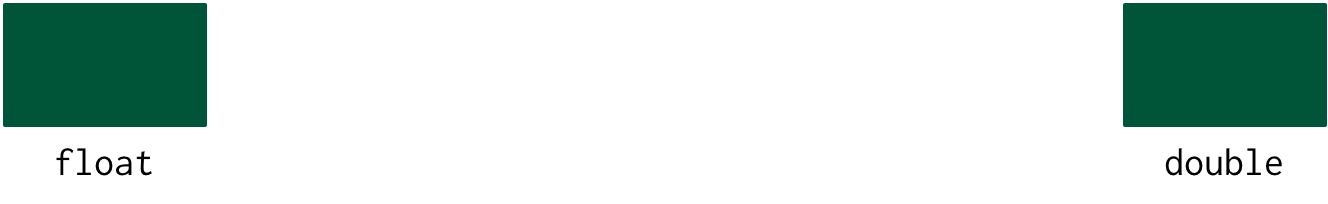There are two sizes of floating point number:

• `float` (32 bit)
• `double` (64 bit, default)

Float literals in xtlang code (e.g. `4.2`) are interpreted as `double` unless the type signatures suggest otherwise (as is the case with `i64` int literals).

### Pointer types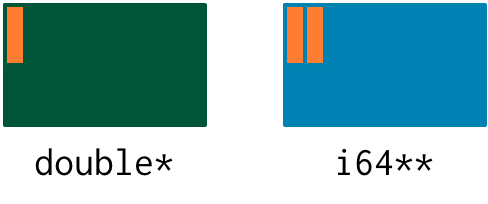xtlang supports pointers to any type, in fact some types (such as closures) are almost always handled by reference (that is, through pointers). Pointers in xtlang are indicated by the usual `*` syntax.

Examples:

• `double*`: a pointer to a double
• `i64**`: a pointer to a pointer to a 64-bit integer

Pointers represent memory addresses, and the use of pointers in xtlang is one of the key differences between xtlang and Scheme (and indeed between xtlang and any high-level language). C programmers will be (intimately) familiar with the concept of pointers, and xtlang’s pointers are the same (you can `printf` them with `%p`) For others, though, a more in-depth explanation of the concept of pointers can be found here <pointer-doc>. If you’ve never encountered pointers before then I suggest you check it out before continuing.

The way to allocate (and store a pointer to) memory is through a call to one of xtlang’s ‘alloc’ functions. Extempore has 3 different alloc functions: `salloc`, `halloc` and `zalloc`. They all allocate a chunk of memory and return a pointer type, but they differ in where that memory is allocated from. In order of how ‘long-lived’ the memory will be: `salloc` allocates memory on the stack (shortest-lived), `zalloc` allocates memory from the current zone, and `halloc` allocates memory from the heap (longest-lived). Finally, `alloc` is an alias for `zalloc`.

More detail about the Extempore’s memory architecture (and the difference between `salloc`, `halloc` and `zalloc`) can be found in memory. For now, though, we’ll just use `zalloc`, which allocates memory from the current zone, which for these examples will work fine.

``````(bind-func ptr_test
(lambda ()
(let ((a:double* (zalloc)))

(ptr_test) ;; prints "address = 0x1163bc030"
``````

In this example, the function closure `ptr_test` takes no arguments, binds a pointer to a `double` (`a`) in the `let`, and then prints the memory address that `a` points to.

Pointers aren’t very interesting, though, if you can’t read and write to the values they point to. That’s where the xtlang functions `pref`, `pset!` and `pref-ptr` come in.

Unlike in C, `*` is not a dereference operator, it’s just the syntax for the specifying pointer types. Instead, there’s a function `pref` for dereferencing a pointer (getting the value the pointer ‘points’ to). `pref` takes two arguments: the pointer, and an (integer) offset. So if `a` is a pointer to a chunk of 10 `double` in memory (such as returned by `zalloc`, for instance), then `(pref a 2)` in xtlang is the value of the third (`pref` uses 0-based indexing) of those `double` (equivalent to `a` in C).

To set the value associated with a pointer, there’s `pset!`. Like `pref`, `pset!` takes a pointer as the first argument, and offset as the second argument, but it also takes an additional third argument—the value to set into that memory location. This must be of the appropriate type: so if the pointer is to a double, then the value passed to `pset!` must also be a double.

``````(bind-func ptr_test2
(lambda ()
(let ((a:double* (zalloc))) ; allocate some memory for a double, bind
; the pointer to the symbol a
(pset! a 0 2.4)          ; set the value at index 0 (of a) to 2.4
(pref a 0))))            ; read the value at index 0 of a

(ptr_test2) ;; returns 2.400000
``````

In this example the closure `ptr_test2` takes no arguments, allocates some memory, sets a value into that memory location, then reads it back out. Notice that for both `pref` and `pset!` the index argument was zero—this means that we were storing and reading the value directly into the pointer (memory location) bound to `a`.

This is important (and useful) because the call to `zalloc` can (optionally) take an integer argument. So, if we know we’re going to store 4 doubles, we can do this:

``````(bind-func ptr_test3
(lambda ()
(let ((a:double* (zalloc 4)))
(pfill! a 1.2 3.4 4.2 1.1) ; fill the pointer a with values
(pref a 2))))              ; read the value at index 2 of a

(ptr_test3) ;; returns 4.200000
``````

`(zalloc 4)` will allocate enough memory for `4` doubles (4 doubles with 64 bytes/double means 256 bytes all up).

There’s one new function in this example: `pfill!`, which is helpful for filling multiple values into a byte array. Using `pfill!` is exactly the same as calling `pset!` 4 times with an index of 0, 1, 2, and 3, but it’s a bit more concise.

Finally, one more useful way to fill values into a chunk of memory is using a loop.

``````(bind-func ptr_test4
(lambda ()
(let ((a:double* (zalloc 10))
(i:i64 0))
(dotimes (i 10)
(pset! a i (i64tod i)))
(pref a 6))))

(ptr_test4) ;; returns 6.000000
``````

There’s one more useful function for working with pointers: `pref-ptr`. Where `(pref a 3)` returns the value of the 4th element of the chunk of memory pointed to by `a`, `(pref-ptr a 3)` returns a pointer to that value. This also implies that `(pref (pref-ptr a n))` is the same as `(pref (pref-ptr a 0) n)` for any integer n.

One final note for C programmers: there is no `void*` in xtlang, use an `i8*` instead.

### C Strings

There’s also no `char` type, just `i8`. So string literals in xtlang are pointers to null terminated arrays (just like in C) but instead have type `i8*`.

The usual `pref` and friends for pointers (described above) are therefore your friends if you want to slice and dice strings around. A few familiar string functions have made their way over from the C standard library as well.

String literals in xtlang are bound globally (allocated on the heap). So you can safely set and store pointers to them without worrying about then disappearing on you.

``````(bind-func string_literals
(lambda ()
(let ((str "Vive le tour!"))
(printf "%s\n" str))))

(string_literals) ;; prints "Vive le tour!"
``````

xtlang does have a nicer `String` type, defined in `libs/base/base.xtm` (TODO more docs coming soon!).

## Aggregate types

There are three base aggregate types in xtlang: tuples, arrays and vectors. In each case, these names mean pretty much the same thing they do in other languages.

Normally the best way to work with these types is by reference (that is, through pointers). Allocating memory for a tuples, array or vector is done through a call to one of the alloc functions, as in the example above with pointers to primitive types.

### Tuples

An n-tuple is a fixed-length structure with n elements. Different tuples can have different lengths (different values of n), but a particular tuple always has the same fixed length.

The elements of a tuple need not be of the same type, tuples are heterogeneous. Each element can be any type that the xtlang compiler recognises, including another tuple—turtles all the way down!

The syntax for declaring and identifying tuples in xtlang is the use of angle brackets (`<>`). Tuples in xtlang are analogous to C structs, except without named members. This is a bit of a pain at the moment, but named structs are high on the Extempore to-do list at the moment and will be added in a future release.

Examples: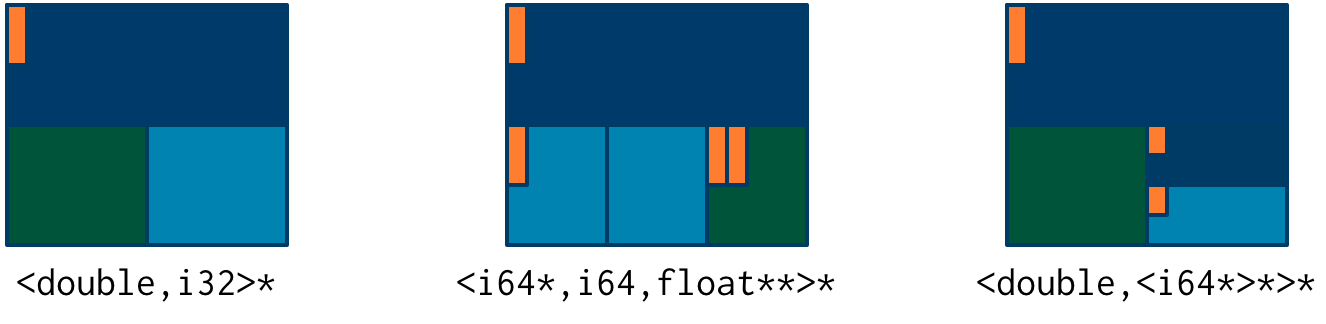• `<double,i32>*` is a pointer to a 2-tuple: the first element is a `double` and the second element is an `i32`
• `<i64*,i64,float**>*` is a pointer to a 3 tuple: the first element is a pointer to an `i64`, the second is an `i64`, and the third is a pointer to a pointer to a `float`
• `<double,<i64*>*>*` is a pointer to a 2-tuple, with a `double` as the first element and a pointer to a 1-tuple as the second

Like `pref` for pointers, getting an element from a tuple involves a function called `tref`. So, to get element number `i` from a tuple pointer `t`, use `(tref t i)`. If `tref` doesn’t have an i th element, the compiler will complain (as it should). The first argument to `tref` should be a pointer to a tuple rather than the tuple itself, and this holds for the array and vector equivalents as well. In fact, you’ll almost never work with aggregate data types directly, instead getting pointers to them via calls to the memory allocation functions. The exception to this rule is if you’re binding to a C library and you need to pass structs around by value (rather than by reference).

Similarly, `tset!` is used to set a value into a tuple. Again, the compiler will check that the value being set is of the right type, and will throw a compile error if there’s a problem.

There’s `tfill!` for filling values into tuples. Again, it’s up to you to make sure that you pass values of the correct types into the different slots. But if you don’t, at least you get a compile time error rather than weird behaviour at runtime.

And finally, if you want a reference to (rather than the value of) an element in the tuple, use `tref-ptr` instead of `tref`.

All of these tuple ref/ref-ptr/set!/fill! functions have the same syntax as the pointer (i.e. `pref`) versions. There are also equivalent functions for arrays (with an `a` prefix) and vectors (`v` prefix). This consistency makes it easier to remember how to work with and access the different types. And because xtlang is strongly typed, even if you do get confused and try to `tset!` an array type the compiler will catch the error for you.

### Vectors

The final aggregate data type in xtlang is the vector type. Vectors are like arrays in that they are fixed length homogeneous type buffers, but operations vector types will use the CPUs SIMD registers and instructions (if your hardware has them). This can potentially give significant speedups for certain types of processing. The downside is that there’s a bit less flexibility (certain operations can’t be performed on vector elements, especially conditionals and branching) and it does make your code a bit less portable, at least from a performance standpoint.

The syntax for vector types looks just like the array syntax, except the pipes (`|`) are replaced with slashes (`/`), presumably because they’re going faster.

Examples: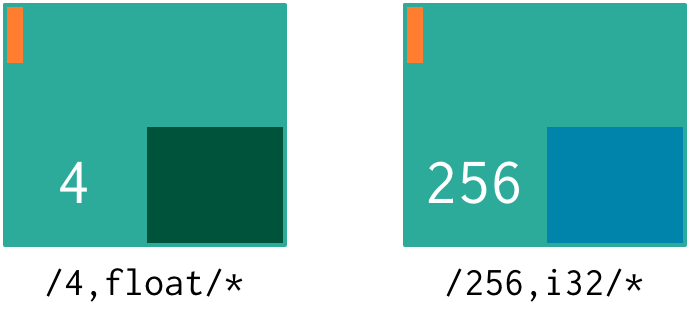• `/4,float/*`: a pointer to a vector of four floats
• `/256,i32/*`: a pointer to a vector of 256 ints

In general, if you’re working with vector types you’ll know what you’re doing, and pick algorithms and word sizes which make good use of the vector hardware on your computer. Unless you know that the particular code you’re working on is the performance bottleneck in your system, it’s probably best to start out with arrays, and to change to vectors later on if it becomes necessary.

### Arrays

An array in xtlang is a fixed length array of elements of a single type (like a static C array). The array type signature specifies the length of the array, the type of the array elements, and is closed off with the pipe (`|`) character.

Examples: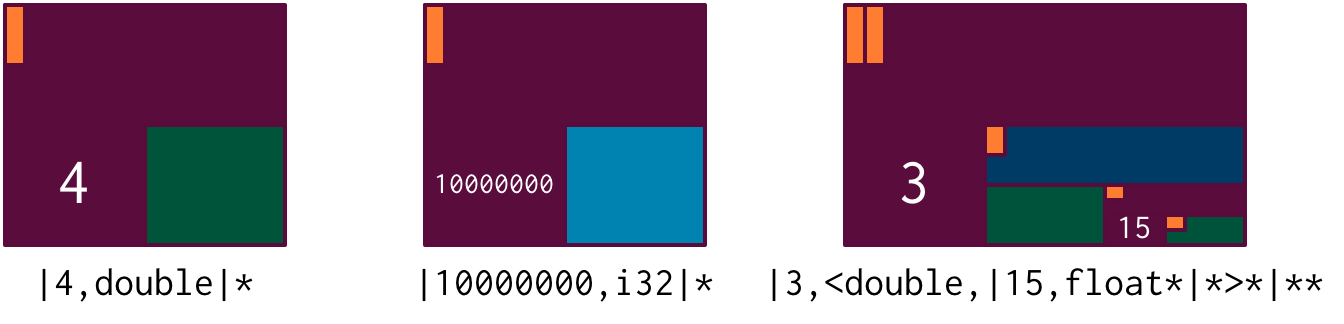• `|4,double|*`: a pointer to an array of 4 `double`
• `|10000000,i32|*`: a pointer to an array of one million `i32`
• `|3,<double,|15,float*|*>*|**`: a pointer to a pointer to an array of pointers to 2-tuples, the second element of which is a pointer to an array of 15 float pointers. Whew!

It’s probably clear at this point that the combinations of types allow for heaps of flexibility, but can get pretty confusing if you use lots of nesting of aggregate types within one another. If you do need to use complex types, then you can define your own types and the compiler can do some of the bookkeeping for you (more on this below).

Setting and getting values from arrays is done with (you guessed it!) `aref`, `aset!`, and `afill!`. And if you want to get a pointer into an array (that is, not to the first element but to some element further into the array), use `aref-ptr`.

### Closures

The final important type in xtlang is the closure type, and understanding closures is crucial to understanding how xtlang works as a whole.

xtlang’s closures are lexical closures (like in Scheme), which means that they are the combination of a function and its referencing environment. This basically means that any variables referred to in the scope of the function (even if they weren’t passed in as arguments) is captured along with the function, and the whole ‘world’ (as far as each little function is concerned) can be passed around in a nice little package.

In xtlang, closure types are indicated by square brackets (`[]`), with the first element inside the brackets being the return type, and any other elements representing the type signature of the function.

Examples: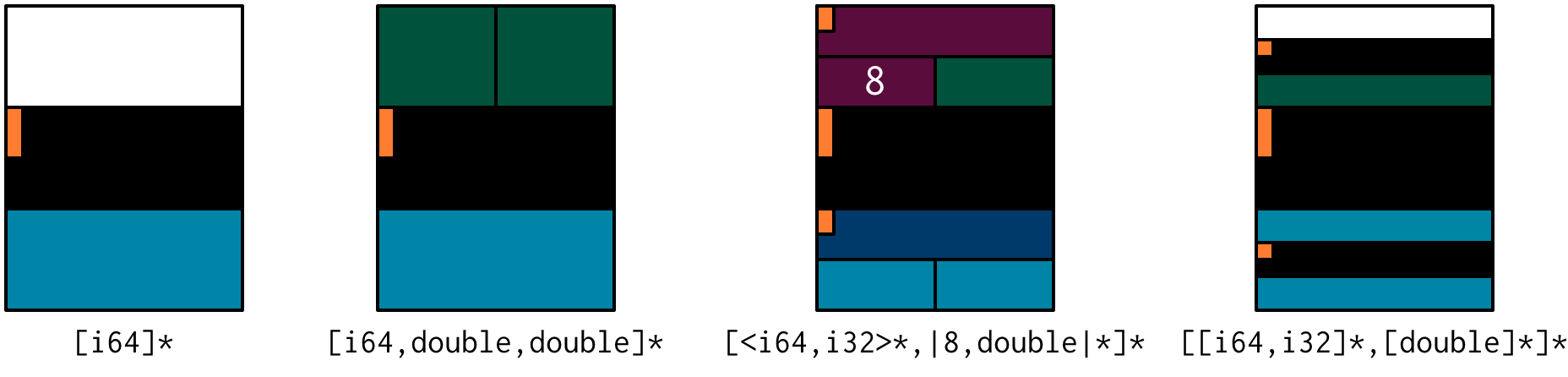• `[i64]*`: a pointer to a closure which takes no arguments and returns a single `i64`
• `[i64,double,double]*`: a pointer to a closure which takes two `double` arguments and returns a single `i64`
• `[<i64,i32>*,|8,double|*]*`: a pointer to a closure which takes as a n argument a pointer to an 8-element `double` array and returns a pointer to a 2-tuple
• `[[i64,i32]*,[double]*]*`: a pointer to a closure which takes a pointer to a closure (which returns a `double`) as an argument and returns a pointer to another closure

The last example in particular is interesting: closures can take closures as arguments, and closures can return other closures. This comes in handy in lots of situations, as lots of the files in Extempore’s `examples` directory show.

The way to make closures in xtlang is with a lambda form, just like in Scheme. A `lambda` returns an anonymous function closure—that’s what it means for xtlang to have ‘first class’ functions/closures. Closures don’t have to have names, they can be elements of lists and arrays, they can be passed to and returned from other closures, they can do anything any other type can do.

Sometimes, though, we want to give a closure a name, and that’s where `bind-func` comes in. `bind-func` is the (only) way in xtlang to assign a global name to a closure. Here’s an example of creating a simple (named) xtlang closure using `bind-func`

``````(bind-func xt_add
(lambda (a:i64 b:i64)
(+ a b)))

(xt_add 3 6) ;; returns 9
``````

`xt_add` takes two int arguments (see how the `i64` type annotations are provided in the initial argument list) and returns their sum. It’s also worth noting that when we compile `xt_add` the log view prints the closure’s type signature:

``````Compiled xt_add >>> [i64,i64,i64]*
``````

`bind-func` is xtlang’s equivalent to Scheme’s `define`, although with the limitation that `define` in Scheme can bind any scheme object (not just a closure) to a symbol, while in xtlang `bind-func` has to return a closure (via a `lambda` form). Although if you need to compile & bind an xtlang entity of some other type, there are other functions like `bind-val` and `bind-type` which will do the necessary for you.

As another example, if you want to return a closure from the function it’s exactly like you would do it in Scheme:

``````(bind-func make_xt_adder
(lambda (a:i64)
(lambda (b:i64)
(+ a b))))

;; type of make_xt_adder is [[i64,i64]*,i64]*

(lambda (c:i64)

;; type of test_xt_adder is [i64,i64]*

``````

This example is a bit more complicated: the first closure (`make_xt_adder`) takes one argument `a` and returns a closure (notice the second `lambda` form inside the toplevel one) which takes one argument `b` and adds it to `a`. Note that `a` is ‘baked in’ to this closure—it’s not passed in directly, but it’s referenced from the outside scope. We say that this closure (which has no name—it’s anonymous) ‘closes over’ `a`.

Then, in the second function (`test_xt_adder`) we call `make_xt_adder` with an argument of 3, so this will return a function closure with one argument which adds 3 to that argument. This (returned) function then gets passed the argument `c` (in this example, it’s called with an argument of 5), so the end result is 3 + 5 = 8. Whew! That’s confusing to read in words, but if you stare at the code long enough you’ll reach enlightenment. Or something.

## Named types

To round it off, you can also define your own types. This is convenient: it’s easier to type `my_type` than `[double*,<i64,i32>,float,float]`, especially if it’s a type that you’ll be using a lot in your code.

There are two ways to define a custom type: `bind-type` and `bind-alias`.

Examples:

``````(bind-alias my_type_1 <i64,double>)
(bind-type my_type_2 <float,[i64,i32]*,|3,double|*>)
``````

`bind-type` tells the xtlang compiler about your new type, which provides some safety benefits: the more the compiler knows about the types in your code, the more errors it can throw at compile time and save messy runtime errors and tricky debugging.

As an example, let’s make a 2D ‘point’ type, and a function for calculating the euclidean distance between two points.

``````(bind-type point <double,double>)

(bind-func euclid_distance
(lambda (a:point* b:point*)
(sqrt (+ (pow (- (tref a 0)
(tref b 0))
2.0)
(pow (- (tref a 1)
(tref b 1))
2.0)))))
``````

To test this out, we can check the diagonal length of the unit square, which should be `sqrt(2) = 1.41`

``````(bind-func test_unit_square_diagonal
(lambda ()
(let ((bot_left:point* (alloc))
(top_right:point* (alloc)))
(tfill! bot_left 0.0 0.0)
(tfill! top_right 1.0 1.0)
(printf "The length of the unit square's diagonal is %f\n"
(euclid_distance bot_left
top_right)))))

(test_unit_square_diagonal)

;; prints "The length of the unit square's diagonal is 1.414214"
``````

Now, what happens if we change this testing example to make `top_right` and `bot_left` just plain tuples of type `<double,double>` instead of being our new `point` type.

``````(bind-func test_unit_square_diagonal_2
(lambda ()
(let ((bot_left:<double,double>* (alloc))
(top_right:<double,double>* (alloc)))
(tfill! bot_left 0.0 0.0)
(tfill! top_right 1.0 1.0)
(printf "The length of the unit square's diagonal is %f\n"
(euclid_distance bot_left
top_right)))))
``````

Now, instead of compiling nicely, we get the compiler error:

``````Compiler Error: Type Error: (euclid_distance bot_left top_right)
function argument does not match. Expected "%point*" but got "{double,double}*"
``````

Even though `point` is just a `<double,double>` (check the `bind-type` definition above), the compiler won’t let us compile the function. This is a good thing most of the time, because it makes us be more explicit about what we actually mean in our code, and saves us from the silly mistakes that can happen when we’re not clear about what we want.

There are lots of possibilities for the use of custom types, and there’s no problem with binding as many as you need to make your code and intention clearer. Binding custom types could, for instance, allow for the construction of a ‘data structures’ library like the C++ STL containers library or the Java collections framework.

`bind-alias`, in contrast to `bind-type`, is just a simple alias for the given type. The xtlang compiler, when it sees `my_alias` in the code, will simply substitute in the appropriate type (in this case `<i64,|3,double|*>*`) before it generates the LLVM IR to send to the compiler. `bind-alias` doesn’t tell the compiler as much about the code as `bind-type` does, which can lead to execution-time problems which would otherwise have been caught by the compiler. So you should almost always use `bind-type` over `bind-alias`.

## Generics

With generics, xtlang provides a way to specify types and closures without concrete types. This allows you to write re-usable code and algorithms for various different types and functions, and allows the compiler to figure out the specific “concrete” types later. This is all still compiled code, and it’s all still very efficient (no boxing/unboxing going on). It’s just nice to not have to write a separate `sort` function for lists of `i32` and `i64` for example.

This section is under construction, but for now have a look in `libs/core/adt.xtm` for Extempore’s abstract data type (ADT) functionality.